46 Writing Good Code
Functions like Table or NestList or FoldList exist in the Wolfram Language because they express common things one wants to do. As in natural language, there are always many ways one can in principle express something. But good code involves finding the most direct and simple way.
Simple and good Wolfram Language code for making a table of the first 10 squares:
 In:=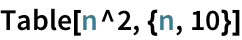Out=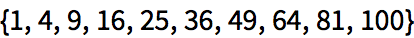Why would anyone write anything else? A common issue is not thinking about the whole table, but instead thinking about the steps in building it. In the early days of computing, computers needed all the help they could get, and there was no choice but to give code that described every step to take.
 In:=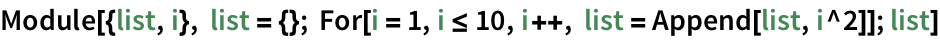Out=But the point of the Wolfram Language is to let one express things at a higher leveland to create code that as directly as possible captures the concept of what one wants to do. Once one knows the language, its vastly more efficient to operate at this level. And it leads to code thats easier for both computers and humans to understand.
 In:=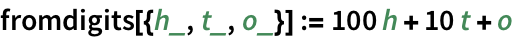Run the code:
 In:=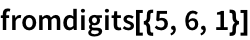Out=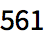In:=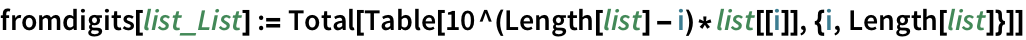The new code works:
 In:=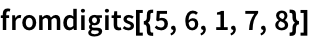Out=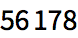Simplify the code by multiplying the whole list of powers of 10 at the same time:
 In:=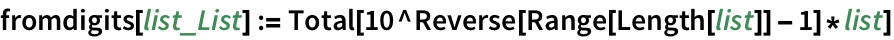In:=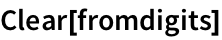In:=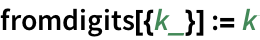In:=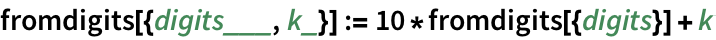The new approach works too:
 In:=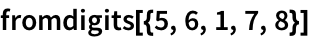Out=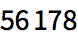In:=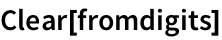In:=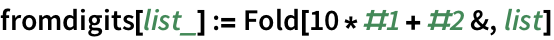In:=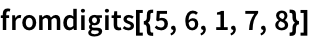Out=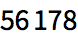In:=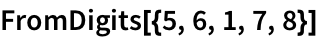Out=In:=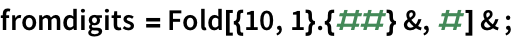It still works though:
 In:=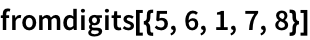Out=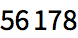Heres a single definition that combines several cases:
 In:=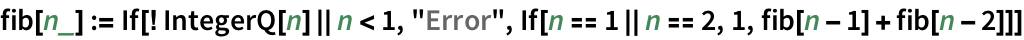In:=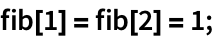In:=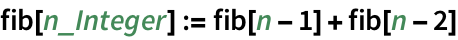When youre writing code, its common to first define a new function because you need it in some very specific context. But its almost always worth trying to give it a name that youll understand even outside that context. And if you cant find a good name, its often a sign that its not quite the right function to define in the first place.
 In:=Out=In:=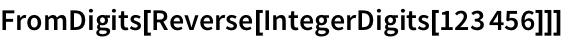Out=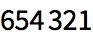In:=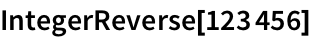Out=With every new version, the Wolfram Language does better at automatically figuring out how to make your code run fast. But you can always help by structuring your algorithms well.
Timing gives the timing of a computation (in seconds), together with its result:
 In:=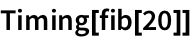Out=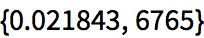With the definitions of fib above, the time grows very rapidly:
 In:=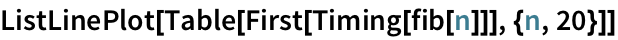Out=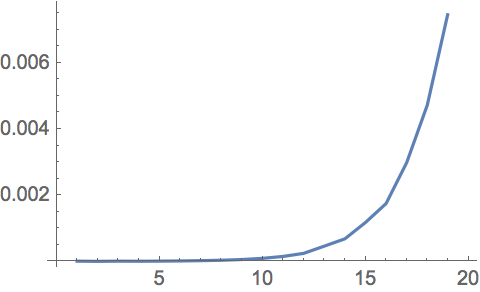Redefine the fib function to remember every value it computes:
 In:=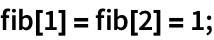In:=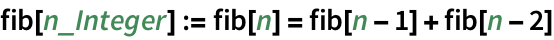Now even up to 1000 each new value takes only microseconds to compute:
 In:=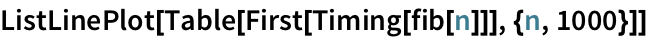Out=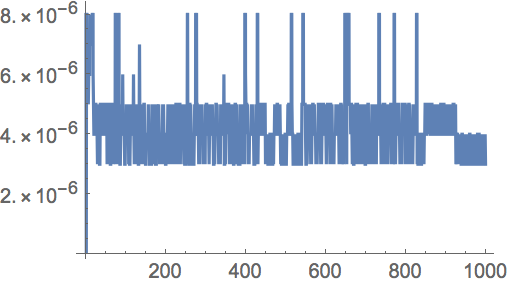FromDigits[list] assemble an integer from its digits IntegerReverse[n] reverse the digits in an integer Timing[expr] do a computation, timing how long it takes
46.1Find a simpler form for Module[{a, i}, a=0; For[i=1, i1000, i++, a=i*(i+1)+a];a]»
Expected output:
 Out[]=Answer & check your solution
46.2Find a simpler form for Module[{a, i}, a=x; For[i=1, i10, i++, a=1/(1+a)];a]»
Expected output:
 Out[]=Answer & check your solution
46.3Find a simpler form for Module[{i, j, a}, a={}; For[i=1, i10, i++, For[j=1, j10, j++, a=Join[a, {i, j}]]];a]»
Expected output:
 Out[]=Answer & check your solution
46.4Make a line plot of the timing for computing n^n for n up to 10000. »
Sample expected output:
 Out[]=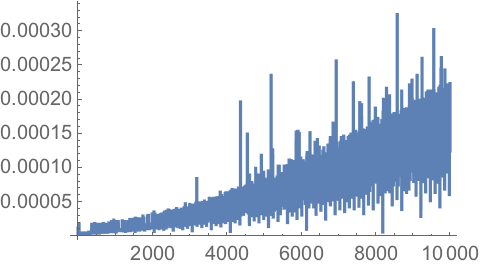Answer & check your solution
46.5Make a line plot of the timing for Sort to sort Range[n] from a random order, for n up to 200. »
Sample expected output:
 Out[]=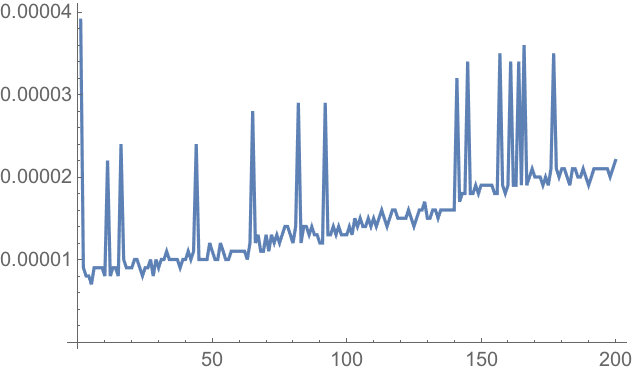Answer & check your solution
Its a short notation for i=i+1. Its the same notation that C and many other low-level computer languages use for this increment operation.
What does the For function do?
Its a direct analog of the for(...) statement in C. For[start, test, step, body] first executes start, then checks test, then executes step, then body. It does this repeatedly until test no longer gives True.
Why can shortened pieces of code be hard to understand?
The most common issue is that variables and sometimes even functions have been factored out, so there are fewer names to read that might give clues about what the code is supposed to do.
Whats the best IDE for authoring Wolfram Language code?
For everyday programming, Wolfram Notebooks are best. Make sure to add sections, text and examples right alongside your code. For large multi-developer software projects, Wolfram Workbench provides an Eclipse-based IDE.
What does Timing actually measure?
Use RepeatedTiming, which runs code many times and averages the timings it gets. (This wont work if the code is modifying itself, like in the last definition of fib above.)
Beyond keeping the code simple, one thing is not to recompute anything you dont have to. Also, if youre dealing with lots of numbers, it may make sense to use N to force the numbers to be approximate. For some internal algorithms you can pick your PerformanceGoal, typically trading off speed and accuracy. There are also functions like Compile that force more of the work associated with optimization to be done up front, rather than during a computation.